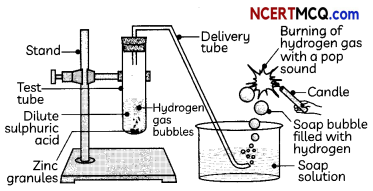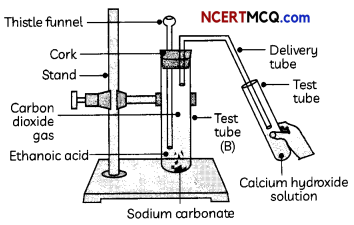## Acids:

On the basis of their chemical properties, all the chemical compounds can be classified into:

• Acids
• Bases
• Salts

In this chapter, we will be studying the various properties of acids and bases, synthetic, natural, and olfactory indicators, behavior of acids and bases in solutions, strength of acids and bases, pH and universal indicator, the importance of pH in everyday life, family of salts, chemicals obtained from common salt, their manufacture, properties and uses, and water of crystallization.

Acids are those chemical substances which have a sour taste and change the colour of blue litmus to red.

## Classifications of Acids:

(A) Based on the source, acids can be classified as mineral acids and organic acids.
Mineral Acids:
The acids prepared from the minerals of the earth are called mineral acids. They are man-made acids. Examples: Hydrochloric acid, sulphuric acid, nitric acid, etc.

Organic Acids:
The acids present in plant materials and animals are called organic acids or naturally occurring acids.

Few examples of naturally occurring acids along with their source are given below:

 Name of Acid Source Lactic Acid Curd Methanoic Acid Insect sting Oxalic Acid Tomato Tartaric Acid Tamarind Citric Acid Citrus fruits such as lemon, oranges, etc. Acetic Acid Vinegar

(B) Based on the degree of dissociation in water, acids can be classified as strong acids and weak acids.
Strong Acids
Acids that give rise to more H+ ions in water are said to be strong acids. In other words, strong acids dissociate completely into hydrogen ions in water. Examples: Hydrochloric acid, sulphuric acid.

Weak Acids:
Acids that are partially ionized in water and give less H+ ions in water are said to be weak acids. In other words, their degree of dissociation into hydrogen ions in water is not much.

Examples: Acetic acid, carbonic acid.## Properties of Acids:

Physical Properties

1. Acids have a sour taste.
2. Acids are soluble in water.
3. Acids turn blue litmus red.
4. Acid solutions conduct electricity.

## Chemical Properties of Acids:

1. Reaction with metals: Acids react with metals to form hydrogen gas. When a metal reacts with an acid, it displaces hydrogen from the acids. The metal combines with the remaining part of the acid and forms a compound called salt.
Acid + Metal → Salt + Hydrogen gas
Zn + H2SO4 → ZnSO4 + H2

Example 1.
Why should curd and sour substances not be kept in brass and copper vessels?
Curd is basically lactic acid. Similarly, sour substances also contain acids. We know that acids react with metals to form salt and water. Therefore, if curd and sour substances are stored in brass and copper vessels, the acid will react with the metal and may produce some salts which are poisonous and hence unfit for human consumption.

2. Reaction with metal carbonates and metal hydrogen carbonates: Acids react with metal carbonates and metal hydrogen carbonates to form carbon dioxide gas.
Metal carbonate/hydrogen carbonate + Acid → Salt + carbon dioxide + water
Na2CO3(S) + 2HCl(aq) → 2NaCl(aq) + H2O(l) + CO2(g)
NaHCO3(s) + HCl(aq) → NaCl(aq) + H2O(l) + CO2(g)

Important
Test for carbon dioxide gas:
When carbon dioxide is passed through lime water, the lime water turns milky due to the formation of a white precipitate of calcium carbonate, CaCO(3)
Ca(OH)2(aq) + CO2(g) → CaCO3(s) + H2O(l)

On passing excess carbon dioxide through lime water, the white precipitate formed first dissolves due to the formation of a soluble salt calcium hydrogen carbonate and the solution becomes clear again.
CaCO3(s) + CO2(g) + H2O(l) → Ca(HCO3)2(aq)Example 2.
A solution reacts with crushed eggshells to give a gas that turns lime-water milky. The solution contains:
(a) NaCl
(b) HCl
(c) Li Cl
(d) KCl
(b) HCl
Explanation: As the gas evolved turns lime water milky, the gas is carbon dioxide. Moreover, the crushed eggshells contain calcium carbonate, which reacts with dilute HCl to give carbon dioxide, salt, and water.

The equation for the reaction taking place is:
CaCO3(s) + 2HCl(aq) → CaCl2(aq) + H2O(aq) + CO2(g)

3. Reaction with bases: Acids react with bases to form salt and water.
Base + Acid → Salt + Water
NaOH(aq) + HCl(aq) → NaCl(aq) + H2O(l)

4. Reaction with metal oxides: Acids react with metal oxides to form salt and water.
Metal Oxide + Acid → Salt + Water
CuO(s) + 2HCl(aq) → CuCl2(aq) + H2O(l)

Example 3.
Case Based:
About 5 ml of dilute sulphuric acid is taken in a test tube ‘A’ and a few pieces of zinc granules are added to it. The gas evolved is passed through the soap solution. The activity is repeated with some more acids like HCl, HNO3 and CH3COOH.Soap bubble filled with hydrogen -Soap solution
Two more test tubes labeled as ‘B’ and ‘C are taken and about 0.5 g of sodium carbonate (Na2CCO3) is taken in test tube ‘B’ and about 0.5 g of sodium hydrogen carbonate (NaHCO) in test tube ‘Cl About 2 mL of dilute HCl is added to both the test tubes. The gas produced in each case is passed through lime water (calcium hydroxide solution).(A) A student recorded his observations on the gas produced in test tubes as:Identify the correct observation:
(d) Test Tube A: hydrogen gas; Test Tube B: Carbon dioxide; Test Tube C: carbon dioxide Explanation: The gas produced in test tube ‘A’ is hydrogen and that in test tubes ‘B’ and ‘C’ is carbon dioxide as the reactions taking place in the test tubes are given below:
Test tube A: Zn + H2SO4 → ZnSCO4 + H2
Test tube ‘B’: Na2CO3(s) + 2HCl(aq) → 2NaCl(aq) + H2O(l) + CO2(g)
Test tube ‘C’: NaHCO3(s) + HCl(aq) → NaCl(aq) + H2O(l) + CO2(g)

(B) Which of the following observations are incorrect?
(I) When the gas evolved in test tube ‘A’ is passed through a soap solution, the soap bubbles formed extinguish a burning candle.
(II) When the gas evolved in test tube ‘A’ is passed through a soap solution, the soap bubbles burn with a pop sound when a burning candle is brought near a bubble.
(III) The gas evolved in test tube ‘B’ turns lime water milky.
(IV) The gas evolved in test tube ‘C’ burns with a pop sound.
(a) Both (I) and (IV)
(b) Both (II) and (III)
(c) (I), (III), and (IV)
(d) (II), (III) and (IV)
(a) Both (I) and (IV)
Explanation: The gas evolved in test tube A’ is hydrogen gas, which burns with a pop sound. The gas evolved in test tubes ‘B’ and ‘C’ is carbon dioxide which turns lime water milky.(C) Are the observations in test tube ‘A’ same when the activity is repeated with more acids like HCl, HNO3 and CH3COOH?
Yes, the same observations are seen when the activity is repeated with more acids like HCl, HNO3, and CH3COOH as metals react with acids and form hydrogen gas by displacing hydrogen atoms from the acid and also forms a salt.
For example, Zn + 2HCl → ZnCl2 + H2

(D) Metal compound A reacts with dilute hydrochloric acid to produce effervescence. The gas evolved extinguishes a burning candle. Write a balanced chemical equation for the reaction if one of the compounds formed is calcium chloride.
As the gas evolved on reacting the metal compound with dilute hydrochloric acid produces effervescence and also extinguishes a burning candle, the gas evolved is carbon dioxide.

Since one of the compounds formed is calcium chloride, metal A is calcium chloride, since metal carbonates react with acid to produce carbon dioxide gas, metal salt, and water.
CaCO3(s) + 2HCl(aq) → CaCl2(aq) + H2O(l) + CO2(g)

(E) Assertion: When an acid reacts with a metal carbonate or metal hydrogen carbonate, the gas evolved turns lime water milky.
Reason: When excess carbon dioxide gas is
passed through lime water, calcium hydrogen carbonate solution is formed.
(a) Both (A) and (R) are true and (R) is the correct explanation of the (A).
(b) Both (A) and (R) are true, but (R) is not the correct explanation of the (A).
(c) (A) is true, but (R) is false.
(d) (A) is false, but (R) is true.